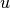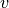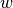Warning

This documents an unmaintained version of NetworkX. Please upgrade to a maintained version and see the current NetworkX documentation.

# within_inter_cluster¶

Compute the ratio of within- and inter-cluster common neighbors of all node pairs in ebunch.

For two nodesand, if a common neighborbelongs to the same community as them,is considered as within-cluster common neighbor ofand. Otherwise, it is considered as inter-cluster common neighbor ofand. The ratio between the size of the set of within- and inter-cluster common neighbors is defined as the WIC measure. 

Parameters: G (graph) – A NetworkX undirected graph. ebunch (iterable of node pairs, optional (default = None)) – The WIC measure will be computed for each pair of nodes given in the iterable. The pairs must be given as 2-tuples (u, v) where u and v are nodes in the graph. If ebunch is None then all non-existent edges in the graph will be used. Default value: None. delta (float, optional (default = 0.001)) – Value to prevent division by zero in case there is no inter-cluster common neighbor between two nodes. See  for details. Default value: 0.001. community (string, optional (default = ‘community’)) – Nodes attribute name containing the community information. G[u][community] identifies which community u belongs to. Each node belongs to at most one community. Default value: ‘community’. piter – An iterator of 3-tuples in the form (u, v, p) where (u, v) is a pair of nodes and p is their WIC measure. iterator

Examples

>>> import networkx as nx
>>> G = nx.Graph()
>>> G.add_edges_from([(0, 1), (0, 2), (0, 3), (1, 4), (2, 4), (3, 4)])
>>> G.node['community'] = 0
>>> G.node['community'] = 1
>>> G.node['community'] = 0
>>> G.node['community'] = 0
>>> G.node['community'] = 0
>>> preds = nx.within_inter_cluster(G, [(0, 4)])
>>> for u, v, p in preds:
...     '(%d, %d) -> %.8f' % (u, v, p)
...
'(0, 4) -> 1.99800200'
>>> preds = nx.within_inter_cluster(G, [(0, 4)], delta=0.5)
>>> for u, v, p in preds:
...     '(%d, %d) -> %.8f' % (u, v, p)
...
'(0, 4) -> 1.33333333'


References

  (1, 2) Jorge Carlos Valverde-Rebaza and Alneu de Andrade Lopes. Link prediction in complex networks based on cluster information. In Proceedings of the 21st Brazilian conference on Advances in Artificial Intelligence (SBIA‘12) http://dx.doi.org/10.1007/978-3-642-34459-6_10